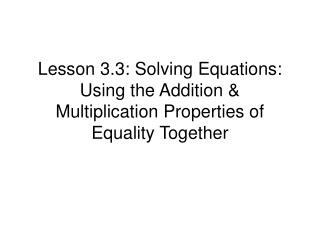DownloadDownload PresentationLesson 3.3: Solving Equations: Using the Addition & Multiplication Properties of Equality Together

# Lesson 3.3: Solving Equations: Using the Addition & Multiplication Properties of Equality Together

Download Presentation## Lesson 3.3: Solving Equations: Using the Addition & Multiplication Properties of Equality Together

- - - - - - - - - - - - - - - - - - - - - - - - - - - E N D - - - - - - - - - - - - - - - - - - - - - - - - - - -
##### Presentation Transcript

1. Lesson 3.3: Solving Equations: Using the Addition & Multiplication Properties of Equality Together

2. REVIEW x + 4 = 7 – 4 – 4 To undo addition you should To undo subtraction you should To undo multiplication you should To undo division you should subtract Addition Property of Equality x – 5 = 3 +5 +5 add 3x = 12 ÷3 ÷3 divide * Multiplication Property of Equality x/6 = 5 *6 *6 multiply *EXCEPTION: When multiplying by a fraction, don’t divide. Instead multiply by the reciprocal. 3/4x = 5 *4/3 *4/3

3. MULTISTEP PROBLEMS Most problems require more than one step to solve. For example: 3x + 4 = 19 coefficient in front of variable something added 3x + 4 = 19 – 4 – 4 3x = 15 Use the Addition Property first to isolate the term with the variable. ÷3 ÷3 x = 5 Then use the Multiplication Property to make the coefficient before the variable be a ‘1’. √ 3*5 + 4 = 19 Always check your answer (plug ‘n chug)! TRY THESE: 1. 5x + 6 = 16 2. –7x + 6 = 13 3. 5 = 4y – 12 4. –4c + 3 = 12

4. MULTISTEP PROBLEMS: SIMPLIFICATION Sometimes problems need to be simplified before solving. Simplification includes: 1) Collecting like terms on the same side of the = sign 2) Distributing through parentheses AFTER these steps are done, you can use the Addition & Multiplication Properties to solve For example: 3x + 7x = 30 Combine them and then solve 3x + 7x = 30 10x = 30 ÷10 ÷10 x = 3 like terms on same side of = sign TRY THESE: 1. 6x + 2x = 16 2. 7x – 3x = 6 3. -2a + 3 + 6a = 14

5. MULTISTEP PROBLEMS: SIMPLIFICATION Sometimes problems need to be simplified before solving. Simplification includes: 1) Collecting like terms on the same side of the = sign 2) Distributing through parentheses AFTER these steps are done, you can use the Addition & Multiplication Properties to solve For example: 2(2y + 3) = 14 Distribute and then solve 2(2y + 3) = 14 4y + 6 = 14 -6 -6 4x = 8 ÷4 ÷4 x = 2 parentheses TRY THESE: 1. 8(3x + 2) = 30 2. 5X +5(4X – 1) = 20